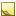﻿ Matrix4x4.CreateFromYawPitchRoll MethodCreates rotation matrix to rotate an object around X, Y and Z axes.

Namespace:  Accord.Math
Assembly:  Accord.Math (in Accord.Math.dll) Version: 3.8.0Syntax
```public static Matrix4x4 CreateFromYawPitchRoll(
float yaw,
float pitch,
float roll
)```

#### Parameters

yaw
Type: SystemSingle
Rotation angle around Y axis in radians.
pitch
Type: SystemSingle
Rotation angle around X axis in radians.
roll
Type: SystemSingle
Rotation angle around Z axis in radians.

#### Return Value

Type: Matrix4x4
Returns rotation matrix to rotate an object around all 3 axes.RemarksNote
The routine assumes roll-pitch-yaw rotation order, when creating rotation matrix, i.e. an object is first rotated around Z axis, then around X axis and finally around Y axis.See Also